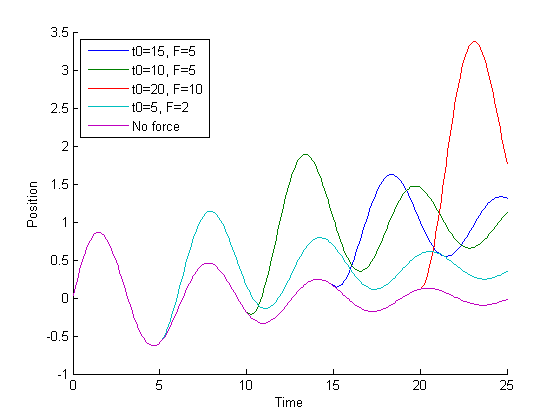# Functional Programming Constructs

Jiro's pick this week is Functional Programming Constructs by Tucker McClure.

Anonymous functions are extremely powerful and useful, and I use them all the time whenever I want to create a quick function without writing a MATLAB file. For me, it's one of those features which the more I use, the more I appreciate. Loren has written this post on anonymous functions, and she has discussed the topic in other posts as well.

For those of you who are not familiar with anonymous functions, let me give you a simple example. Tapping into my mechanical engineering background, let's simulate a damped harmonic oscillator by solving the second-order differential equation numerically with ode45.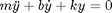We solve the ODE by passing in the system of first order differential equations in the form of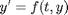A second order differential equation becomes a system of two first order differential equations.

function dY = odeFcn(t, y, m, k, b)
dY = [y(2); -1/m * (k * y(1) + b * y(2))];
end

Then you can solve the system like this (after defining m, k, b, and T):

[~, Y] = ode45(@(t, y) odeFcn(t, y, m, k, b), T, [0, 1]);

Simple enough. However, instead of creating a separate function file for odeFcn, I can just create an anonymous function. I can do this because this is a simple, one-statement function.

% Define mass, spring stiffness, and damping
m = 5; k = 5; b = 1;

% Create anonymous function for the damped harmonic oscillator
odeFcnAnon = @(t, y) [y(2); -1/m * (k * y(1) + b * y(2))];

Now, we can just pass the function handle (to the anonymous function) odeFcnAnon to ode45:

% Define time vector
T = 0:0.1:25;

% Solve
[~, Y] = ode45(odeFcnAnon, T, [0, 1]);
plot(T, Y);
legend('Position', 'Velocity');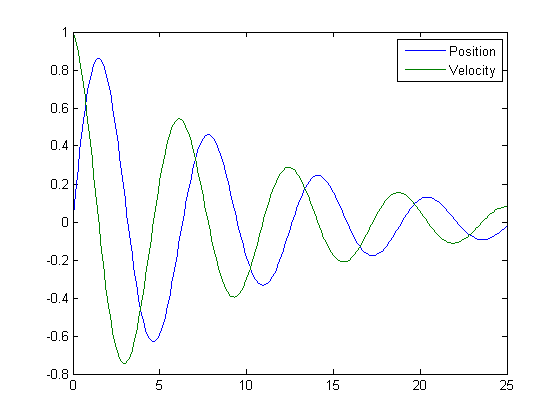Wasn't that great? I didn't have to write a separate file or a function. I just created the ODE function "on the fly". However, I can't create any function on the fly. An anonymous function can only contain one executable statement, i.e. I cannot have multiple statements or flow control commands like if and for.

Well, that's a bummer! I wanted to make my dampled oscillator behave a little differently by adding an external force at a certain time. To do that, my ODE function would look something like this:

function dY = odeFcn(t, y, m, k, b, t0, F)
if t > t0  % after time t0, apply force F
dY = [y(2); -1/m * (k * y(1) + b * y(2) - F)];
else
dY = [y(2); -1/m * (k * y(1) + b * y(2))];
end
end

This certainly has more than one statement. How can I possibly do this with an anonymous function? Is my only choice to write a separate function?? ...

Not anymore! My colleague, Tucker, comes to the rescue! He has created a number of functions for flow control commands, as well as other functions that aide in use inside anonymous functions. For example, he has a function iif, which is the functional form of if.

iif(<if this>,      <then that>, ...
<else if this>, <then that>, ...
<else>,         <then that>);

Let's see what our ODE anonymous function would look like with this.

odeFcnAnon = @(t, y, t0, F) iif( ...
t > t0, @() [y(2); -1/m*(k*y(1)+b*y(2)-F)], ... % if t > t0
true  , @() [y(2); -1/m*(k*y(1)+b*y(2)  )]);    % else

And, let's solve. Notice that I'm passing in two extra arguments, t0 and F.

t0 = 15;   % Force applied at 15 seconds
F = 5;     % External force
[~, Y] = ode45(@(t, y) odeFcnAnon(t, y, t0, F), T, [0, 1]);
plot(T, Y);
legend('Position', 'Velocity');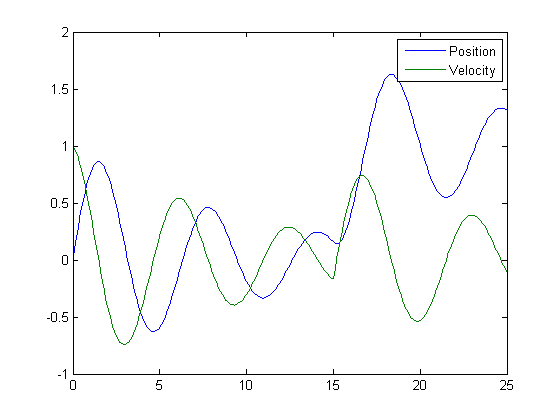That was cool. I was able to create an anonymous function for my function that contained conditional statements.

There are lots more to Tucker's functions, and he has written a detailed document deriving some of those functions step by step. It's well-written for such a complex topic. I highly recommend reading through it.

Let me show a couple of more functions, and I'll leave the rest for you to explore. One of the things that are not possible with anonymous functions is the ability to deal with outputs of functions. For example, inside an anonymous function, I am not able to access the second output of a function, or index into the first column of the output. This is because I can't have multiple statements inside an anonymous function, like this:

@() [var1, var2] = myFunction;var2(:, 1)

For this, Tucker has created output and paren. Let's create an anonymous function that would solve the ODE, extract the position vector, and plot it.

plotODEFcn = @(t0, F) plot(T, ...
paren(...
output(...
@() ode45(@(t,y) odeFcnAnon(t,y,t0,F), T, [0, 1]), ...
2), ...   % Get the 2nd output from ODE45  -  Y
':', 1));        % Get the 1st column of Y        -  Y(:, 1)

A little confusing? Don't worry. I was confused as well at first. If you read Tucker's documentation, you'll get a better understanding.

Now, we can easily create multiple plots with different forcing conditions.

figure; hold all;
plotODEFcn(15, 5);   % Force of 5 at 15 seconds
plotODEFcn(10, 5);   % Force of 5 at 10 seconds
plotODEFcn(20, 10);  % Force of 10 at 20 seconds
plotODEFcn(5, 2);    % Force of 2 at 5 seconds
plotODEFcn(0, 0);    % No external force

xlabel('Time'); ylabel('Position');
legend('t0=15, F=5', 't0=10, F=5', 't0=20, F=10', 't0=5, F=2', 'No force', ...
'Location', 'NorthWest')